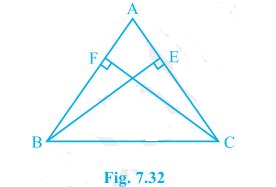# ABC is a triangle in which altitudes BE and CF to sides AC and AB are equal (see Fig.) . Show that (i) ΔABE ΔACF (ii) AB = AC, i.e., ABC is an isosceles triangle.#### Solution

Given

Altitudes BE and CF to sides AC and AB are equal

To Prove

(i) ΔABE ≅ ΔACF

(ii) AB = AC

Proof

(i) ΔABE ≅ ΔACF

In ∆ABF and ∆ACF,

∠E=∠F [Each 90° angle]

∠A=∠A [common angle]

AB=AC [given] S

∴∆AEB≅∆AFC [A.A.S]

(ii) AB=AC [C.P.C.T]

Hence Proved(32)(7)